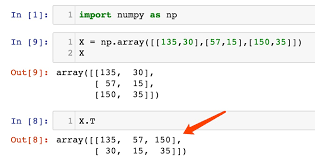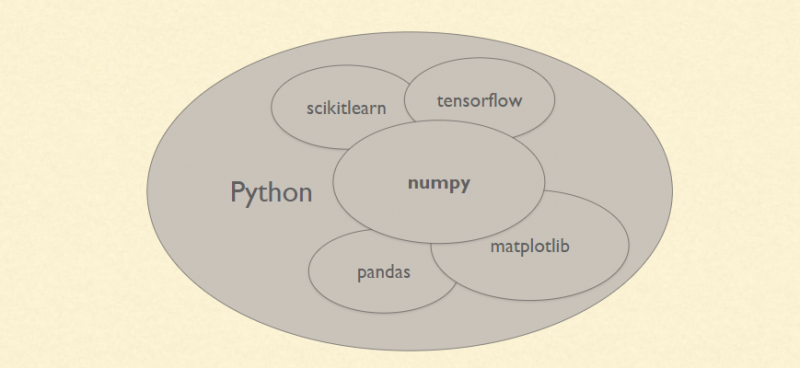# Pandas Tutorial- How to run analysis using numeric python (numpy) with example

## Numpy and Pandas

Numpy is a python package that is used for scientific computing. It provides support for large multi-dimensional arrays and matrices. Pandas is a python library used for data manipulation and analysis. Having a solid knowledge of both libraries is extremely useful for feature engineering, data imputation, and model building

#### >>> import numpy as npSome of the important attributes of a NumPy object are:

1. Ndim: displays the dimension of the array
2. Shape: returns a tuple of integers indicating the size of the array
3. Size: returns the total number of elements in the NumPy array
4. Dtype: returns the type of elements in the array, i.e., int64, character
5. Itemsize: returns the size in bytes of each item
6. Reshape: Reshapes the NumPy array
``` # list
bob =[1,2,3]
print(bob)
import numpy as np

# In:

# 1-Dimensional array
tuna =[1,3,4,5,6,7,8]
np.array(tuna)

# In:
# 2-Dimensional array
mat = ([1,2,3],[4,5,6],[5,6,7])
np.array(mat)

# In:
#note that when dealing with 2-D
# it always end with 2 ']]' of that
# and if it is three of it ']]]' then it is 3-D

# In:
np.arange(0,11)

# In:

# when dealing with range in array we use arange
np.arange(0,11,2) # the last parameter is the step(even num)

# In:

# means 9 dot(.)
np.zeros(9)+2

# In:

np.zeros((2,4)) # 2 -&amp;amp;amp;amp;gt; num of rows
# 4 -&amp;amp;amp;amp;gt; num of columns

# In:

np.ones((2,5))

# In[ ]:

# In:

# linspace will take the number of POINT(dot. ) that we want e.g below is 5
np.linspace(0,5,5)

# In:

#creating identity matrix
# it a 2-D -&amp;amp;amp;amp;gt; number of rows = number of colums
np.eye(5, dtype=int)

# In:

#the random library can also be used by
from numpy.random import randint
randint(2,7) #it give a random num from btw 2-&amp;amp;amp;amp;gt;6

# In:

# arrays of numbers = 1-D
np.random.rand(5)

# In:

np.random.rand(5,5) # 2-D

# In:

np.random.randn(4,2) #return num &amp;amp;amp;amp;lt; 0

# In:

# it give a random num btw the number but exclusive 100
np.random.randint(0,100)

# In:

np.random.randint(56,100,10)
#10 means 10 random numbers will be displayed butexclusive 100

# In:

#attribute of an array
arr = np.arange(25)
arr

# In:

# reshape is used to return the data of an array
# e.g arr above is 25 element and can be RESHAPED as 5x5 = 25
arr.reshape(5,5)

# In:

rar = np.random.randint(0,51,10)
rar

# In:

rar.reshape(2,5)

# In:

#to return the maximum and minimum of an array
rar

# In:

rar.max()

# In:

rar.min()

# In:

#getting the index location of the max value
rar.argmax()

# In:

#getting the index location of the min value
rar.argmin()

# In:

#getting the shape of a vector = size of array (1-D)
rar.shape

# In:

rar  = rar.reshape(2,5)
rar.shape

# In:

#getting datatype
arr.dtype

# In:

rar.dtype

# In:

np.full((5,4),3.14)

# In:

mat = np.arange(1,26).reshape(5,5)
mat

# In:

# NB all counting start from 0 for both rows and cloumn
mat[2:] # count row 2 down

# In:

mat[2:,1:] # 1: reps column

# In:

mat[3:,3:]

```#### >>> import pandas as pd

Some commonly used data structures in pandas are:

1. Series objects: 1D array, similar to a column in a spreadsheet
2. DataFrame objects: 2D table, similar to a spreadsheet
3. Panel objects: Dictionary of DataFrames, similar to sheet in MS Excel
```# In:
import pandas as pd
# In:
import numpy as np
# In:
labs = ['a','b','c']
my_data = [11,30,40]
arr = np.array(my_data)
d = { 'a': 20, 'b':30,'c':40}
# In:
pd.Series(data = my_data)
# In:
pd.Series(data = my_data, index=labs)
# In:
#OR
pd.Series(my_data,labs)
# In:
pd.Series(arr,d)
# In:
pd.Series(d)
# In:
# a pandas series can hold a varities of numbers or object types
# In:
ser1 = pd.Series([1,2,3,4],['USA','Germany','USSR','Japan'])
ser1
# In:
ser2 = pd.Series([1,2,6,4],['USA','Germany','Italy','Japan'])
# In:
#passing the index of 1
ser1['USA']
# In:
ser3 = pd.Series(labs, my_data)
ser3
# In:
ser3 #index labs
# In:
ser1
# In:
ser2
# In:
ser1 + ser2  #integer is converted to float
# In[ ]:
```